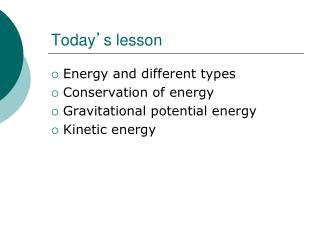Download PresentationToday ’ s lessonToday ’ s lesson - PowerPoint PPT Presentation

Download PresentationToday ’ s lesson
An Image/Link below is provided (as is) to download presentation

Download Policy: Content on the Website is provided to you AS IS for your information and personal use and may not be sold / licensed / shared on other websites without getting consent from its author. While downloading, if for some reason you are not able to download a presentation, the publisher may have deleted the file from their server.

- - - - - - - - - - - - - - - - - - - - - - - - - - - E N D - - - - - - - - - - - - - - - - - - - - - - - - - - -
Presentation Transcript

1. Today’s lesson • Energy and different types • Conservation of energy • Gravitational potential energy • Kinetic energy

2. Energy Rules • Energy is needed to make stationary objects move, to change their shape or to warm them up. When you life an object, you transfer energy from your muscles to the object!

3. Forms of Energy • Potential energy : energy due to position • Kinetic energy: energy due to motion • Thermal energy: energy due to the temperature of an object • Chemical or nuclear energy: energy associated with chemical or nuclear reactions. • Electrical energy: energy of electrically charged objects • Elastic energy: energy stored in an object when it is stretched or compressed.

4. Units for Energy • Energy is measured in Joules (J) • 1 Joule = energy needed to raise a 1N weight through a vertical height of 1m

5. REMEMBER!!! • Energy can be changed form one form into other forms… • The total energy amount after the change is ALWAYS equal to the total amount of energy before the change. • ENERGY CANNOT BE CREATED OR DESTROYED

6. POTENTIAL ENERGY • ENERGY of an object due to its position.

7. Chemical kinetic gravitational Gain in GPE = work done = m x g x Δh

8. ΔEp = mgΔh m kg Joules N/kg or m/s2

9. Example Woof! (help!) A dog of mass 12 kg falls from an aeroplane at a height of 3.4 km. How much gravitational energy does the dog lose as it falls to the ground

10. Example On earth g = 10 m/s2 Mass of dog = 12 kg Height = 3.4 km = 3400 m

11. Example On earth g = 10 m/s2 Mass of dog = 12 kg GPE lost by dog = mgh = 12 x 10 x 3400 = 408 000 J Height = 3.4 km = 3400 m

12. Example GPE lost by dog = mgh = 12 x 10 x 3400 = 408 000 J Just before the dog hits the ground, what has this GPE turned into?

13. Kinetic energy

14. Kinetic energy Kinetic energy of an object can be found using the following formula Ek = mv2 2 where m = mass (in kg) and v = speed (in m/s)

15. Example A bullet of mass 150 g is travelling at 400 m/s. How much kinetic energy does it have?

16. Example A bullet of mass 150 g is travelling at 400 m/s. How much kinetic energy does it have? Ek = mv2/2 = (0.15 x (400)2)/2 = 12 000 J

17. Energy changes

18. Let’s try some questions!